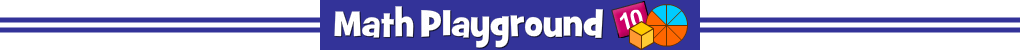Complete the number puzzle using both positive and negative integers.
The number in each block is the sum of the two numbers directly underneath.
Number Conundrum Integers - Learning Connections
Essential Skills
Mental Math - combine numbers to make target sums

Common Core Connection for Grades 4-6
Positive and negative numbers describe quantities having opposite directions or values.
Opposite signs of numbers indicate locations on opposite sides of 0 on a number line.
Position integers on a horizontal or vertical number line diagram.
Understand ordering and absolute value of rational numbers.
More Math Games to Play